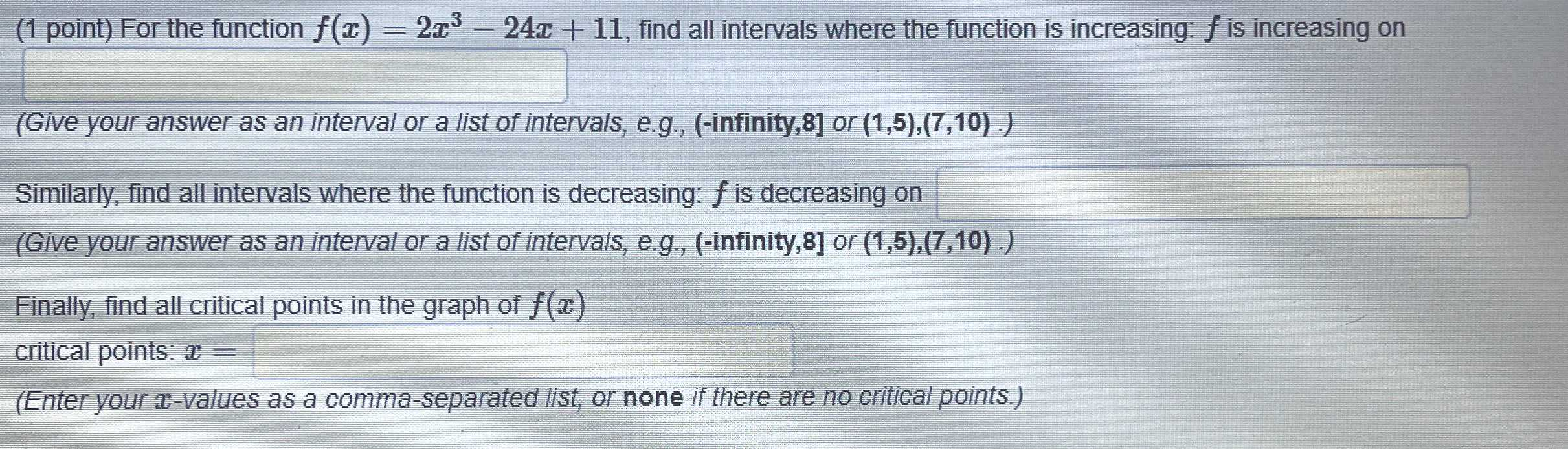### ¿Todavía tienes preguntas de matemáticas?

Pregunte a nuestros tutores expertos
Algebra
Pregunta(1 point) For the function $$f ( x ) = 2 x ^ { 3 } - 24 x + 11$$ , find all intervals where the function is increasing: $$f$$ is increasing on

(Give your answer as an interval or a list of intervals, e.g., $$($$ -infinity, $$8 ]$$ or $$( 1,5 ) , ( 7,10 ) . )$$

Similarly, find all intervals where the function is decreasing: $$f$$ is decreasing on

(Give your answer as an interval or a list of intervals, e.g., $$($$ -infinity, $$8 ]$$ or $$( 1,5 ) , ( 7,10 ) . )$$

Finally, find all critical points in the graph of $$f ( x )$$

critical points: $$x =$$

(Enter your $$x$$ -values as a comma-separated list, or none if there are no critical points.)

$$(- \infty ,- 2)\cup (2,+ \infty )$$